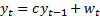/

### Extreme Events – Specimen Question A.4.1(a) – Answer/Hints

Q. Estimate the value of c 16 times, the first time assuming that you only have access to the first 5 observations, the next time you only have access to the first 6 observations, etc.

In each estimation, the value of c can be estimated as the slope of the best fit line in a standard linear regression (e.g. using the function SLOPE in Microsoft Excel or the MnSlope Nematrian web function). [N.B. Some refinement of this would be needed if the model were the simpler onesince this is equivalent to the constrained regression where the line of best fit is forced to pass through the origin.]

Using logged returns, these values of c are:

 Estimation up to and including period Estimated value of c 5 -0.3354190 6 -0.4051569 7 -0.3408570 8 -0.3844843 9 0.0505541 10 0.1635878 11 0.1143870 12 -0.0408473 13 0.2354016 14 0.3187719 15 0.3548701 16 0.3061011 17 0.3293394 18 0.3233795 19 0.3163910 20 0.3218388